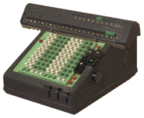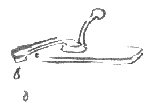# Home CalculationsIf my lawn sprinkler puts out five gallons of water per minute and covers an area 20 feet in diameter, how long should I water to get the equivalent of 1 inch of rain?
```pi(10 feet)^2; 5 gal/min; 1 inch ? minutes
= 39.1679 minutes
```How long does it take for your water heater to recover after you've taken a long hot shower?

Assuming you have a 40 gallon 5000 watt electric water heater, set at 140 degrees, and that water comes into the house at 45 degrees (Fahrenheit)...
Note: shv_water is defined as: 1 cal/cc deltaC

```40 gal; shv_water; (140-45)deltaF; 5000 watt ? hour
= 1.85881 hour
```
What's with the semicolons?You might also ask what it costs to take that shower?

```5000 watt; 1.8 hours; 5 cents/kWh ? \$
= 0.45 \$
```Or how long for a 40000 btu/hour gas heater to recover?
```40 gal; shv_water; 95 deltaF; 40000 btu/hr ? min
= 47.5686 min
```How energy efficient is a window fan?
Assume the inside temperature is 85 degrees F, the outside temp is 60 degrees F and the fan moves 100 cubic feet per minute using 60 watts of electrical power.
```(85 - 60) deltaF * shv_air * 100 cfm ? ton_refrig
= 0.24212 ton_refrig

0.24212 ton_refrig ? watt
= 851.504 watt

851.504 watt / 60 watt ? %
= 1419.17 %
```
That's a pretty good energy efficiency factor!How "HOT" is my microwave?

Measured: It takes 2 min 4 sec to bring 3/4 cup of water from 53 degF to boiling.

```(3/4)cup; shv_water; (212 - 52)deltaF; 2 min + 4 sec ? watts
= 532.552 watts
```What prop pitch is required for a boat to do 40 knots at 2500 rpm?
Assume a 10 % slip
```40 knot / (2500 rpm * 90 %) ? inch/rev
= 21.604 inch/rev
```How much power lost by a leaky hot water faucet and what's it cost?

First measure how big the leak is, say it drips out 1/4 cup in 1 min 49 sec and the water heater is set at 130 deg F. (water comes in at 50 deg F)

```(130-50)deltaF; shv_water; (1/4)cup; 1 min + 49 sec ? watt
= 100.973 watt

100 watt; 6 cents/kWh ? \$/year
= 52.56 \$/year
```How long before water from a faucet flows hot?

Assume the pipe connecting a faucet to the water heater is 43 feet long, has an internal diameter of 1/2 inch, and that the flow rate is 3 gallons per minute.

```43 feet; pi((1/4)inch)^2; 3 gal/min ? sec
= 8.77197 sec
```

Calchemy Software Inc.
Fort Collins, COLast Updated 6/21/2009